# Theoretical Metal Weight Calculation Formula (30 Types of Metals)

If you’re working in the metalworking industry, even you’re engineers, you will try to find one online calculator to help you calculate the weight of various metals and steels including ms plate, gi sheet, structural steel, ms angle, mild steel, steel bar, square tube, angle, aluminum etc.

Then below steel weight calculator and metal weight calculator to calculate the weight of metals in various shapes will be helpful for you.

However, sometimes you may want to learn the weight calculation formula and keep it in your mind, here is it:

Weight (kg) = Sectional Area (mm2) × Length (m) × Density (ρ, g/cm3)× 1/1000

For the metal density, you can also refer to:

From the above two posts, you will find the density of various metals and alloys.

In order to help you quickly find the metal weight formula, I made infographic which is not only practical but also beautiful. (To the bottom)

P.S: Among all of the metal weight calculation formula in the following part, there are two kinds of metals that are not mentioned, which are I beam steel and H beam steel.

If you want to know the difference between H beam steel and I beam steel as well as the methods to calculate the weight of H beam steel and I beam steel, you will get the answers from the following two quick links:

## Theoretical Steel Weight Chart

If you want to get the weight of various metals from the chart, you can refer to the following page:### Steel Plate

• Formula: length(m)×width(m)×thickness(mm)×7.85
• E.g: 6m (length)×1.51m(width)×9.75mm (thickness)
• Calculation: 6×1.51×9.75×7.85=693.43kg### Steel Pipe

• Formula: (OD-wall thickness)×wall thickness(mm)×length(m)×0.02466
• E.g: 114mm(OD)×4mm(wall thickness)×6m(length)
• Calculation: (114-4)×4×6×0.02466=65.102kg### Steel Rod

• Formula: dia.(mm)×dia.(mm)×length(m)×0.00617
• E.g: Φ20mm (dia.)×6m(length)
• Calculation: 20×20×6×0.00617=14.808kg### Square Steel

• Formula: side width(mm)×side width(mm)×length(m)×0.00785
• E.g: 50mm(side width)×6m(length)
• Calculation: 50×50×6×0.00785=117.75(kg)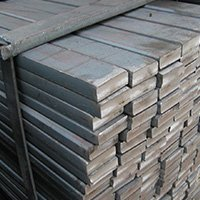### Flat Steel

• Formula: side width(mm)×thickness(mm)×length(m)×0.00785
• E.g: 50mm(side width)×5.0mm(thickness)×6m(length)
• Calculation: 50×5×6×0.00785=11.7.75(kg)### Hexagonal Steel

• Formula: side-to-side dia.×side-to-side dia.× length(m)×0.0068
• E.g: 50mm(dia.)×6m (length)
• Calculation: 50×50×6×0.0068=102(kg)### Rebar

• Formula: dia.mm×dia.mm×length(m)×0.00617
• E.g: Φ20mm(dia.)×12m(length)
• Calculation: 20×20×12×0.00617=29.616kg### Flat Steel Tube

• Formula: (side length+side width)×2×thickness×length(m)×0.00785
• E.g: 100mm×50mm×5mm(thickness)×6m (length)
• Calculation: (100+50)×2×5×6×0.00785=70.65kg### Rectangular Steel Tube

• Formula: side width(mm)×4×thickness×length(m)×0.00785
• E.g: 50mm×5mm (thickness)×6m(length)
• Calculation: 50×4×5×6×0.00785=47.1kg### Equal-leg Angle Steel

• Formula: (side width×2-thickness)×thickness×length(m)×0.00785
• E.g: 50mm×50mm×5(thickness)×6m(length)
• Calculation: (50×2-5)×5×6×0.00785=22.37kg### Unequal-leg Angle Steel

• Formula: (side width+side width-thickness)×thickness×length(m)×0.0076
• E.g: 100mm×80mm×8(thickness)×6m(length)
• Calculation: (100+80-8)×8×6×0.0076=62.746kg### Brass Pipe

• Formula: (OD-wall thickness)×thickness(mm)×length(m)×0.0267### Copper Pipe

• Formula: (OD-wall thickness)×thickness(mm)×length(m)×0.02796### Aluminum Checkered Sheet

• Formula: length(m)×width(mm)×thickness (mm)×0.00296### Brass Pipe

• Formula: thickness(mm)×(O.D-thickness)×length(m)×0.0267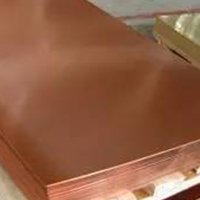### Copper Sheet

• Formula: length(m)×width(mm)×thickness(mm)×0.0089### Zinc Plate

• Formula: length(m)×width(mm)×thickness(mm)×0.0072• Formula: length(m)×width(mm)×thickness(mm)×0.01137### Octagonal Steel

• Formula: length(m)×across width(mm)×across width(mm)×0.0065### Copper Rod

• Formula: dia.(mm)×dia.(mm)×length(m)×0.00698### Brass Rod

• Formula: dia.(mm)×dia.(mm)×length(m)×0.00668### Aluminum Rod

• Formula: dia.(mm)×dia.(mm)×length(m)×0.0022### Square Copper Rod

• Formula: width(mm)×width(mm)×length(m)×0.0089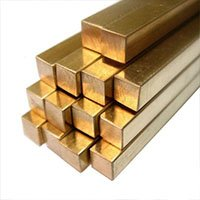### Square Brass Rod

• Formula: width(mm)×width(mm)×length(m)×0.0085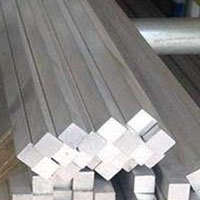### Square Aluminum Rod

• Formula: width(mm)×width(mm)×length(m)×0.0028### Hexagonal Copper Rod

• Formula: across width(mm)×across width(mm)×length(m)×0.0077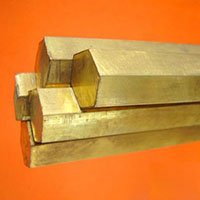### Hexagonal Brass Rod

• Formula: width(mm)×across width(mm)×length(m)×0.00736### Hexagonal Aluminum Rod

• Formula: across width(mm)×across width(mm)×length(m)×0.00242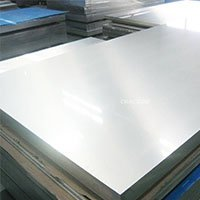### Aluminum Plate

• Formula: thickness(mm)×width(mm)×length(m)×0.00171### Aluminum Pipe

• Formula: thickness(mm)×(O.D(mm)-thickness(mm))×length(m)×0.00879